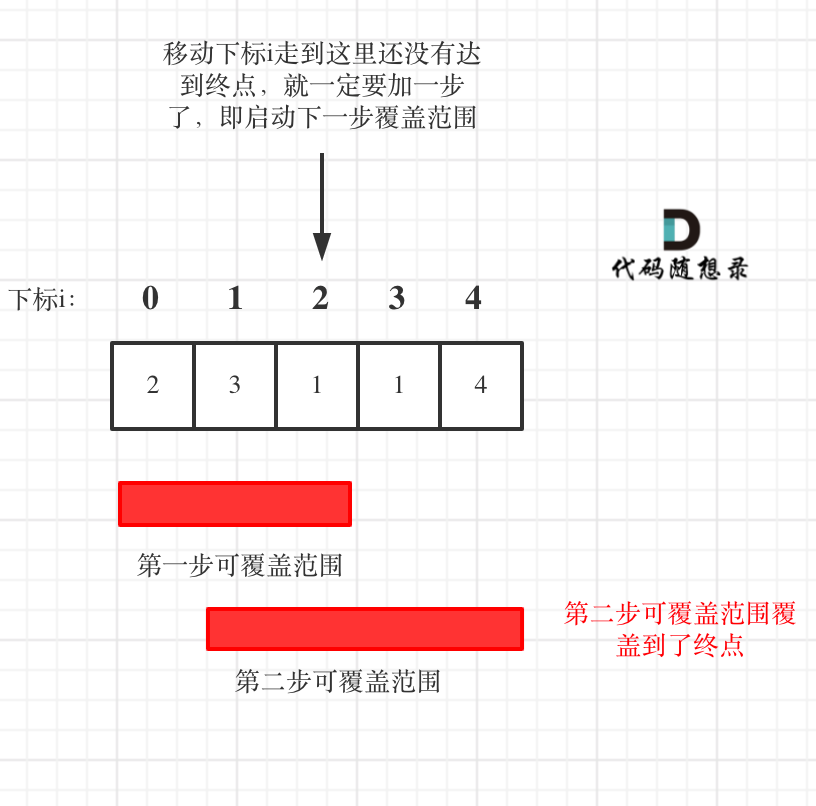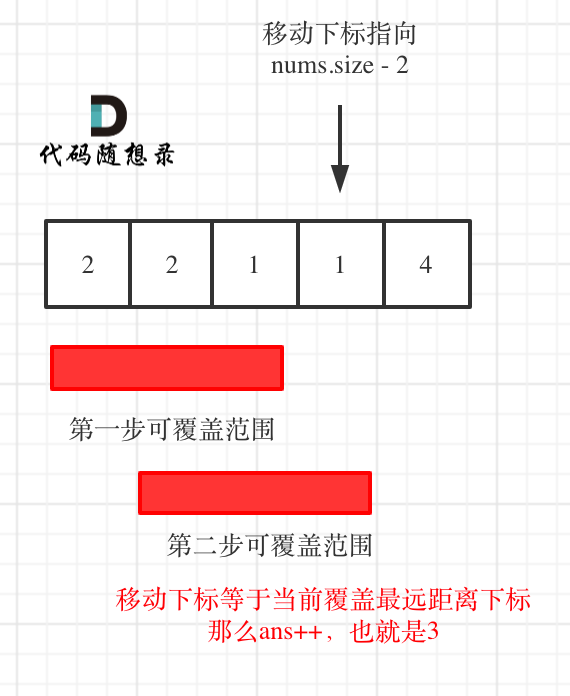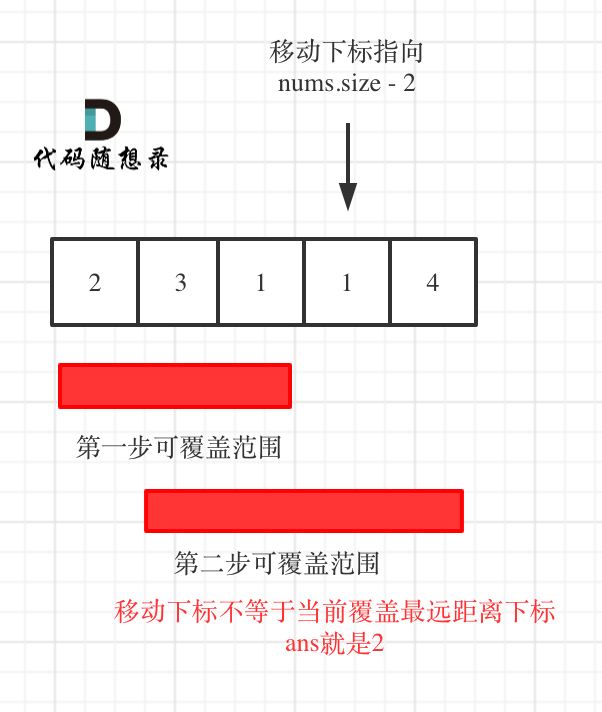# 45.跳跃游戏II

• 输入: [2,3,1,1,4]
• 输出: 2
• 解释: 跳到最后一个位置的最小跳跃数是 2。从下标为 0 跳到下标为 1 的位置，跳 1 步，然后跳 3 步到达数组的最后一个位置。

# 思路# 方法一

• 如果当前覆盖最远距离下标不是是集合终点，步数就加一，还需要继续走。
• 如果当前覆盖最远距离下标就是是集合终点，步数不用加一，因为不能再往后走了。

C++代码如下：（详细注释）

// 版本一
class Solution {
public:
int jump(vector<int>& nums) {
if (nums.size() == 1) return 0;
int curDistance = 0;    // 当前覆盖最远距离下标
int ans = 0;            // 记录走的最大步数
int nextDistance = 0;   // 下一步覆盖最远距离下标
for (int i = 0; i < nums.size(); i++) {
nextDistance = max(nums[i] + i, nextDistance);  // 更新下一步覆盖最远距离下标
if (i == curDistance) {                         // 遇到当前覆盖最远距离下标
if (curDistance != nums.size() - 1) {       // 如果当前覆盖最远距离下标不是终点
ans++;                                  // 需要走下一步
curDistance = nextDistance;             // 更新当前覆盖最远距离下标（相当于加油了）
if (nextDistance >= nums.size() - 1) break; // 下一步的覆盖范围已经可以达到终点，结束循环
} else break;                               // 当前覆盖最远距离下标是集合终点，不用做ans++操作了，直接结束
}
}
return ans;
}
};
1
2
3
4
5
6
7
8
9
10
11
12
13
14
15
16
17
18
19
20
21

# 方法二

• 如果移动下标等于当前覆盖最大距离下标， 需要再走一步（即ans++），因为最后一步一定是可以到的终点。（题目假设总是可以到达数组的最后一个位置），如图：• 如果移动下标不等于当前覆盖最大距离下标，说明当前覆盖最远距离就可以直接达到终点了，不需要再走一步。如图：// 版本二
class Solution {
public:
int jump(vector<int>& nums) {
int curDistance = 0;    // 当前覆盖的最远距离下标
int ans = 0;            // 记录走的最大步数
int nextDistance = 0;   // 下一步覆盖的最远距离下标
for (int i = 0; i < nums.size() - 1; i++) { // 注意这里是小于nums.size() - 1，这是关键所在
nextDistance = max(nums[i] + i, nextDistance); // 更新下一步覆盖的最远距离下标
if (i == curDistance) {                 // 遇到当前覆盖的最远距离下标
curDistance = nextDistance;         // 更新当前覆盖的最远距离下标
ans++;
}
}
return ans;
}
};
1
2
3
4
5
6
7
8
9
10
11
12
13
14
15
16
17

# 其他语言版本

# Java

class Solution {
public int jump(int[] nums) {
if (nums == null || nums.length == 0 || nums.length == 1) {
return 0;
}
//记录跳跃的次数
int count=0;
//当前的覆盖最大区域
int curDistance = 0;
//最大的覆盖区域
int maxDistance = 0;
for (int i = 0; i < nums.length; i++) {
//在可覆盖区域内更新最大的覆盖区域
maxDistance = Math.max(maxDistance,i+nums[i]);
//说明当前一步，再跳一步就到达了末尾
if (maxDistance>=nums.length-1){
count++;
break;
}
//走到当前覆盖的最大区域时，更新下一步可达的最大区域
if (i==curDistance){
curDistance = maxDistance;
count++;
}
}
return count;
}
}
1
2
3
4
5
6
7
8
9
10
11
12
13
14
15
16
17
18
19
20
21
22
23
24
25
26
27
28

# Python

class Solution:
def jump(self, nums: List[int]) -> int:
if len(nums) == 1: return 0
ans = 0
curDistance = 0
nextDistance = 0
for i in range(len(nums)):
nextDistance = max(i + nums[i], nextDistance)
if i == curDistance:
if curDistance != len(nums) - 1:
ans += 1
curDistance = nextDistance
if nextDistance >= len(nums) - 1: break
return ans
1
2
3
4
5
6
7
8
9
10
11
12
13
14

# Go

func jump(nums []int) int {
dp:=make([]int ,len(nums))
dp=0

for i:=1;i<len(nums);i++{
dp[i]=i
for j:=0;j<i;j++{
if nums[j]+j>i{
dp[i]=min(dp[j]+1,dp[i])
}
}
}
return dp[len(nums)-1]
}
1
2
3
4
5
6
7
8
9
10
11
12
13
14

# Javascript

var jump = function(nums) {
let curIndex = 0
let nextIndex = 0
let steps = 0
for(let i = 0; i < nums.length - 1; i++) {
nextIndex = Math.max(nums[i] + i, nextIndex)
if(i === curIndex) {
curIndex = nextIndex
steps++
}
}

return steps
};
1
2
3
4
5
6
7
8
9
10
11
12
13
14@2021-2022 代码随想录 版权所有 粤ICP备19156078号-3